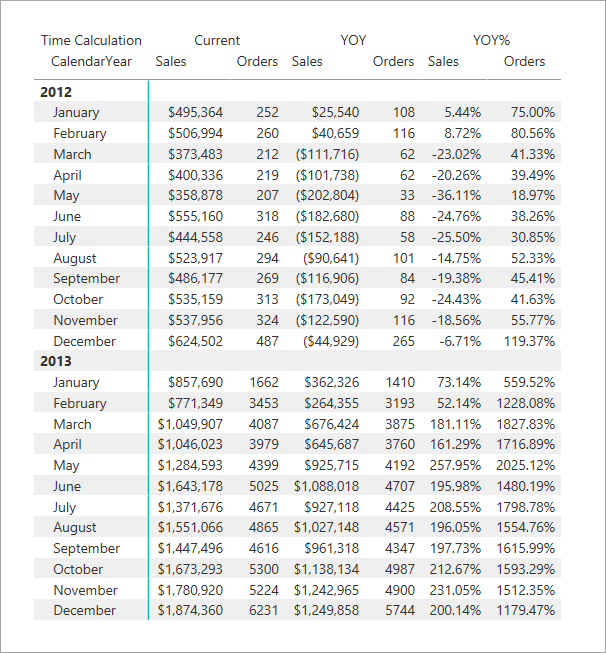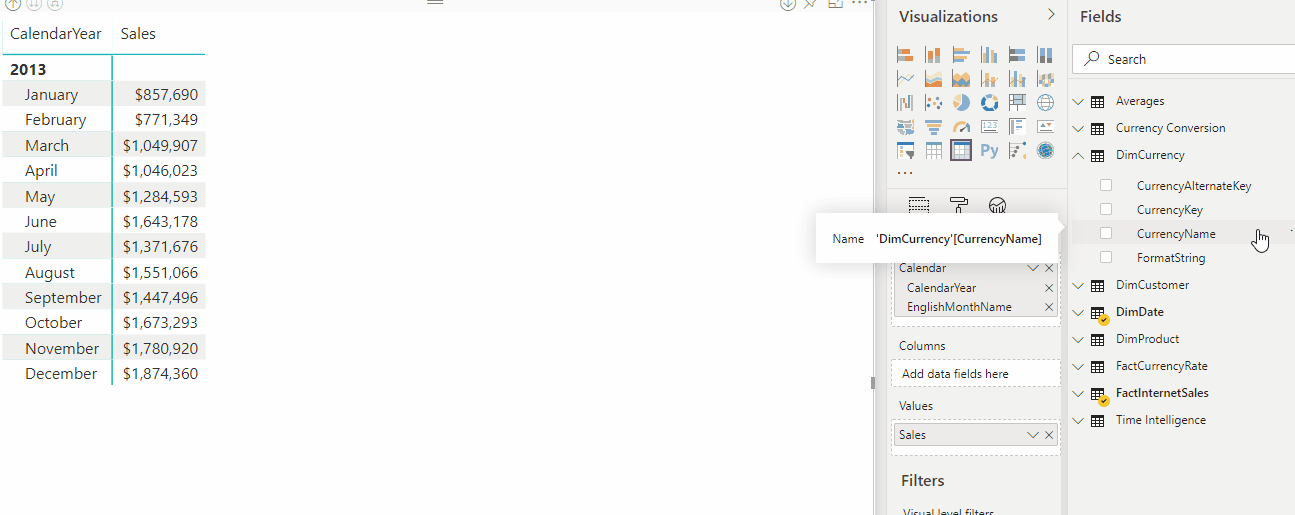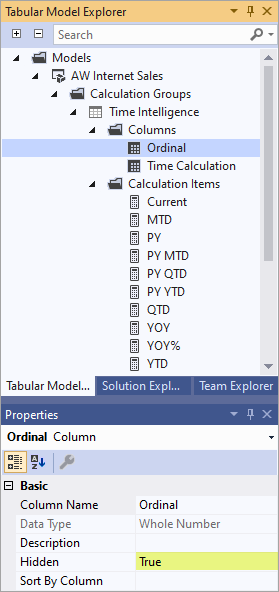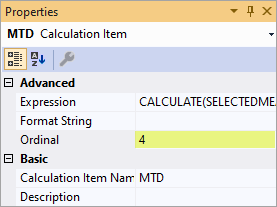# Calculation groups

Applies to:SQL Server 2019 and later Analysis ServicesAzure Analysis ServicesPower BI Premium

Calculation groups can significantly reduce the number of redundant measures by grouping common measure expressions as calculation items. Calculation groups are supported in tabular models at the 1500 and higher compatibility level.

• Benefits
• How calculation groups work
• Dynamic format strings
• Precedence
• Sideways recursion
• Ordering
• How to create
• Limitations

## Benefits

Calculation groups address an issue in complex models where there can be a proliferation of redundant measures using the same calculations - most common with time intelligence calculations. For example, a sales analyst wants to view sales totals and orders by month-to-date (MTD), quarter-to-date (QTD), year-to-date (YTD), orders year-to-date for the previous year (PY), and so on. The data modeler has to create separate measures for each calculation, which can lead to dozens of measures. For the user, this can mean having to sort through just as many measures, and apply them individually to their report.

Let's first take a look at how calculation groups appear to users in a reporting tool like Power BI. We'll then take a look at what makes up a calculation group, and how they're created in a model.

Calculation groups are shown in reporting clients as a table with a single column. The column isn't like a typical column or dimension, instead it represents one or more reusable calculations, or calculation items that can be applied to any measure already added to the Values filter for a visualization.

In the following animation, a user is analyzing sales data for years 2012 and 2013. Before applying a calculation group, the common base measure Sales calculates a sum of total sales for each month. The user then wants to apply time intelligence calculations to get sales totals for month to date, quarter to date, year to date, and so on. Without calculation groups, the user would have to select individual time intelligence measures.

With a calculation group, in this example named Time Intelligence, when the user drags the Time Calculation item to the Columns filter area, each calculation item appears as a separate column. Values for each row are calculated from the base measure, Sales.Calculation groups work with explicit DAX measures. In this example, Sales is an explicit measure already created in the model. Calculation groups do not work with implicit DAX measures. For example, in Power BI implicit measures are created when a user drags columns onto visuals to view aggregated values, without creating an explicit measure. At this time, Power BI generates DAX for implicit measures written as inline DAX calculations - meaning implicit measures cannot work with calculation groups. A new model property visible in the Tabular Object Model (TOM) has been introduced, DiscourageImplicitMeasures. Currently, in order to create calculation groups this property must be set to true. When set to true, Power BI Desktop in Live Connect mode disables creation of implicit measures.

Calculation groups also support Multidimensional Data Expressions (MDX) queries. This means, Microsoft Excel users, which query tabular data models by using MDX, can take full advantage of calculation groups in worksheet PivotTables and charts.

## How they work

Now that you've seen how calculation groups benefit users, let's take a look at how the Time Intelligence calculation group example shown is created.

Before we go into the details, let's introduce some new DAX functions specifically for calculation groups:

SELECTEDMEASURE - Used by expressions for calculation items to reference the measure that is currently in context. In this example, the Sales measure.

SELECTEDMEASURENAME - Used by expressions for calculation items to determine the measure that is in context by name.

ISSELECTEDMEASURE - Used by expressions for calculation items to determine the measure that is in context is specified in a list of measures.

SELECTEDMEASUREFORMATSTRING - Used by expressions for calculation items to retrieve the format string of the measure that is in context.

### Time Intelligence example

Table name - Time Intelligence
Column name - Time Calculation
Precedence - 20

#### Time Intelligence calculation items

Current

``````SELECTEDMEASURE()
``````

MTD

``````CALCULATE(SELECTEDMEASURE(), DATESMTD(DimDate[Date]))
``````

QTD

``````CALCULATE(SELECTEDMEASURE(), DATESQTD(DimDate[Date]))
``````

YTD

``````CALCULATE(SELECTEDMEASURE(), DATESYTD(DimDate[Date]))
``````

PY

``````CALCULATE(SELECTEDMEASURE(), SAMEPERIODLASTYEAR(DimDate[Date]))
``````

PY MTD

``````CALCULATE(
SELECTEDMEASURE(),
SAMEPERIODLASTYEAR(DimDate[Date]),
'Time Intelligence'[Time Calculation] = "MTD"
)
``````

PY QTD

``````CALCULATE(
SELECTEDMEASURE(),
SAMEPERIODLASTYEAR(DimDate[Date]),
'Time Intelligence'[Time Calculation] = "QTD"
)
``````

PY YTD

``````CALCULATE(
SELECTEDMEASURE(),
SAMEPERIODLASTYEAR(DimDate[Date]),
'Time Intelligence'[Time Calculation] = "YTD"
)
``````

YOY

``````SELECTEDMEASURE() -
CALCULATE(
SELECTEDMEASURE(),
'Time Intelligence'[Time Calculation] = "PY"
)
``````

YOY%

``````DIVIDE(
CALCULATE(
SELECTEDMEASURE(),
'Time Intelligence'[Time Calculation]="YOY"
),
CALCULATE(
SELECTEDMEASURE(),
'Time Intelligence'[Time Calculation]="PY"
)
)
``````

To test this calculation group, execute a DAX query in SSMS or the open-source DAX Studio. Note: YOY and YOY% are omitted from this query example.

#### Time Intelligence query

``````EVALUATE
CALCULATETABLE (
SUMMARIZECOLUMNS (
DimDate[CalendarYear],
DimDate[EnglishMonthName],
"Current", CALCULATE ( [Sales], 'Time Intelligence'[Time Calculation] = "Current" ),
"QTD",     CALCULATE ( [Sales], 'Time Intelligence'[Time Calculation] = "QTD" ),
"YTD",     CALCULATE ( [Sales], 'Time Intelligence'[Time Calculation] = "YTD" ),
"PY",      CALCULATE ( [Sales], 'Time Intelligence'[Time Calculation] = "PY" ),
"PY QTD",  CALCULATE ( [Sales], 'Time Intelligence'[Time Calculation] = "PY QTD" ),
"PY YTD",  CALCULATE ( [Sales], 'Time Intelligence'[Time Calculation] = "PY YTD" )
),
DimDate[CalendarYear] IN { 2012, 2013 }
)
``````

#### Time Intelligence query return

The return table shows calculations for each calculation item applied. For example, see QTD for March 2012 is the sum of January, February and March 2012.## Dynamic format strings

Dynamic format strings with calculation groups allow conditional application of format strings to measures without forcing them to return strings.

Tabular models support dynamic formatting of measures by using DAX's FORMAT function. However, the FORMAT function has the disadvantage of returning a string, forcing measures that would otherwise be numeric to also be returned as a string. This can have some limitations, such as not working with most Power BI visuals depending on numeric values, like charts.

### Dynamic format strings for time intelligence

If we look at the Time Intelligence example shown above, all the calculation items except YOY% should use the format of the current measure in context. For example, YTD calculated on the Sales base measure should be currency. If this were a calculation group for something like an Orders base measure, the format would be numeric. YOY%, however, should be a percentage regardless of the format of the base measure.

For YOY%, we can override the format string by setting the format string expression property to 0.00%;-0.00%;0.00%. To learn more about format string expression properties, see MDX Cell Properties - FORMAT STRING Contents.

In this matrix visual in Power BI, you see Sales Current/YOY and Orders Current/YOY retain their respective base measure format strings. Sales YOY% and Orders YOY%, however, overrides the format string to use percentage format.### Dynamic format strings for currency conversion

Dynamic format strings provide easy currency conversion. Consider the following Adventure Works data model. It's modeled for one-to-many currency conversion as defined by Conversion types.A FormatString column is added to the DimCurrency table and populated with format strings for the respective currencies.For this example, the following calculation group is then defined as:

### Currency Conversion example

Table name - Currency Conversion
Column name - Conversion Calculation
Precedence - 5

#### Calculation items for Currency Conversion

No Conversion

``````SELECTEDMEASURE()
``````

Converted Currency

``````IF(
//Check one currency in context & not US Dollar, which is the pivot currency:
SELECTEDVALUE( DimCurrency[CurrencyName], "US Dollar" ) = "US Dollar",
SELECTEDMEASURE(),
SUMX(
VALUES(DimDate[Date]),
CALCULATE( DIVIDE( SELECTEDMEASURE(), MAX(FactCurrencyRate[EndOfDayRate]) ) )
)
)
``````

Format string expression

``````SELECTEDVALUE(
DimCurrency[FormatString],
SELECTEDMEASUREFORMATSTRING()
)
``````

The format string expression must return a scalar string. It uses the new SELECTEDMEASUREFORMATSTRING function to revert to the base measure format string if there are multiple currencies in filter context.

The following animation shows the dynamic format currency conversion of the Sales measure in a report.## Precedence

Precedence is a property defined for a calculation group. It specifies the order of evaluation when there is more than one calculation group. A higher number indicates greater precedence, meaning it will be evaluated before calculation groups with lower precedence.

For this example, we'll use same model as the time intelligence example above, but also add an Averages calculation group. The Averages calculation group contains average calculations that are independent of traditional time intelligence in that they don't change the date filter context - they just apply average calculations within it.

In this example, a daily average calculation is defined. Calculations such as average barrels of oil per day are common in oil-and-gas applications. Other common business examples include store sales average in retail.

While such calculations are calculated independently of time intelligence calculations, there may well be a requirement to combine them. For example, a user might want to see barrels of oil per day YTD to view the daily oil rate from the beginning of the year to the current date. In this scenario, precedence should be set for calculation items.

### Averages example

Table name is Averages.
Column name is Average Calculation.
Precedence is 10.

#### Calculation items for Averages

No Average

``````SELECTEDMEASURE()
``````

Daily Average

``````DIVIDE(SELECTEDMEASURE(), COUNTROWS(DimDate))
``````

Here's an example of a DAX query and return table:

#### Averages query

``````EVALUATE
CALCULATETABLE (
SUMMARIZECOLUMNS (
DimDate[CalendarYear],
DimDate[EnglishMonthName],
"Sales", CALCULATE (
[Sales],
'Time Intelligence'[Time Calculation] = "Current",
'Averages'[Average Calculation] = "No Average"
),
"YTD", CALCULATE (
[Sales],
'Time Intelligence'[Time Calculation] = "YTD",
'Averages'[Average Calculation] = "No Average"
),
"Daily Average", CALCULATE (
[Sales],
'Time Intelligence'[Time Calculation] = "Current",
'Averages'[Average Calculation] = "Daily Average"
),
"YTD Daily Average", CALCULATE (
[Sales],
'Time Intelligence'[Time Calculation] = "YTD",
'Averages'[Average Calculation] = "Daily Average"
)
),
DimDate[CalendarYear] = 2012
)
``````

#### Averages query returnThe following table shows how the March 2012 values are calculated.

Column name Calculation
YTD Sum of Sales for Jan, Feb, Mar 2012
= 495,364 + 506,994 + 373,483
Daily Average Sales for Mar 2012 divided by # of days in March
= 373,483 / 31
YTD Daily Average YTD for Mar 2012 divided by # of days in Jan, Feb, and Mar
= 1,375,841 / (31 + 29 + 31)

Here's the definition of the YTD calculation item, applied with precedence of 20.

``````CALCULATE(SELECTEDMEASURE(), DATESYTD(DimDate[Date]))
``````

Here's Daily Average, applied with a precedence of 10.

``````DIVIDE(SELECTEDMEASURE(), COUNTROWS(DimDate))
``````

Since the precedence of the Time Intelligence calculation group is higher than that of the Averages calculation group, it's applied as broadly as possible. The YTD Daily Average calculation applies YTD to both the numerator and the denominator (count of days) of the daily average calculation.

This is equivalent to the following expression:

``````CALCULATE(DIVIDE(SELECTEDMEASURE(), COUNTROWS(DimDate)), DATESYTD(DimDate[Date]))
``````

Not this expression:

``````DIVIDE(CALCULATE(SELECTEDMEASURE(), DATESYTD(DimDate[Date])), COUNTROWS(DimDate)))
``````

## Sideways recursion

In the Time Intelligence example above, some of the calculation items refer to others in the same calculation group. This is called sideways recursion. For example, YOY% references both YOY and PY.

``````DIVIDE(
CALCULATE(
SELECTEDMEASURE(),
'Time Intelligence'[Time Calculation]="YOY"
),
CALCULATE(
SELECTEDMEASURE(),
'Time Intelligence'[Time Calculation]="PY"
)
)
``````

In this case, both expressions are evaluated separately because they are using different calculate statements. Other types of recursion are not supported.

## Single calculation item in filter context

In our Time Intelligence example, the PY YTD calculation item has a single calculate expression:

``````CALCULATE(
SELECTEDMEASURE(),
SAMEPERIODLASTYEAR(DimDate[Date]),
'Time Intelligence'[Time Calculation] = "YTD"
)
``````

The YTD argument to the CALCULATE() function overrides the filter context to reuse the logic already defined in the YTD calculation item. It's not possible to apply both PY and YTD in a single evaluation. Calculation groups are only applied if a single calculation item from the calculation group is in filter context.

## Ordering

By default, when a column from a calculation group is placed in a report, calculation items are ordered alphabetically by name in the report. The order in which calculation items appear in a report can be changed by specifying the Ordinal property. Specifying calculation item order with the Ordinal property does not change precedence, the order in which calculation items are evaluated. It also does not change the order in which calculation items appear in Tabular Model Explorer.

To specify the ordinal property for calculation items, you must add a second column to the calculation group. Unlike the default column where Data Type is Text, a second column used for ordering calculation items has a Whole Number data type. The only purpose for this column is to specify the numeric order in which calculation items in the calculation group appear. Because this column provides no value in a report, it's best to set the Hidden property to True.After a second column is added to the calculation group, you can specify the Ordinal property value for calculation items you want to order.## Create a calculation group

Calculation groups are supported in Visual Studio with Analysis Services Projects VSIX update 2.9.2 and later. Calculation groups can also be created by using Tabular Model Scripting Language (TMSL) or the open source Tabular Editor.

### To create a calculation group by using Visual Studio

1. In Tabular Model Explorer, right-click Calculation Groups, and then click New Calculation Group. By default, a new calculation group will have a single column and a single calculation item.

2. Use Properties to change the name and enter a description for the calculation group, column, and default calculation item.

3. To enter a DAX formula expression for the default calculation item, right-click and then click Edit Formula to open DAX Editor. Enter a valid expression.

4. To add additional calculation items, right-click Calculation Items, and then click New Calculation Item.

### To order calculation items

1. In Tabular Model Explorer, right-click a calculation group, and then click Add column.

2. Name the column Ordinal (or something similar), enter a description, and then set the Hidden property to True.

3. For each calculation item you want to order, set the Ordinal property to a positive number. Each number is sequential, for example, a calculation item with an Ordinal property of 1 will appear first, a property of 2 will appear second, and so on. Calculation items with the default -1 are not included in the ordering, but will appear before ordered items in a report.

## Limitations

Object level security (OLS) defined on calculation group tables is not supported. However, OLS can be defined on other tables in the same model. If a calculation item refers to an OLS secured object, a generic error is returned.

Row level security (RLS) is not supported. Define RLS on tables in the same model, but not on calculation groups themselves (directly or indirectly).

Detail Rows Expressions are not supported with calculation groups.

Smart narrative visuals in Power BI are not supported with calculation groups.

Implicit column aggregations in Power BI are not supported for models with calculation groups. Currently, if DiscourageImplicitMeasures property is set to false (default), aggregation options appear, however they cannot be applied. If DiscourageImplicitMeasures is set to true, aggregation options do not appear.

When creating Power BI reports using LiveConnection, Dynamic format strings are not applied to report-level measures.